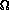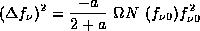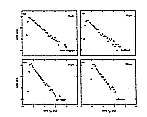VIII.C. Point Source Confusion

# IRAS Explanatory Supplement VIII. Sky Coverage, Confusion, Completeness and Reliability C. Point Source Confusion

Chapter Contents | Authors | References

It is the aim of this section to show that confusion caused by background fluctuations due to the large numbers of sources below the detection threshold is insignificant in the IRAS point source catalog outside of the Galactic plane and other high source density areas.

Suppose that the mean density N(fv) of sources on the sky with flux density greater than fv obeys a power law:

N(fv) = K × fva

(VIII.C.1)

where a (<0) is a constant. In a small interval in flux density (fv, fv + dfv) and within a beam of solid angleone expects on averagea N(fv) fv-1 dfv sources. The actual number will be a stochastic variable with a mean-squared deviation equal to the average value. The mean-squared deviation in the flux will be fv2 × the deviation in the number. Thus the mean-squared flux deviation, (fv)2, from all sources below some threshold fv0 is given by(VIII.C.2)

Equation (VIII.C.2) can be used to estimate the effect of confusion on the IRAS survey in two ways. first, the confusion noise can be calculated directly from the equation using the observed density and distribution of sources. Differential sources counts, dN/d(log fv), are given in Fig. VIII.C.1a-d for sources in all wavelength bands with |b| > 50°. The plots demonstrate that at high Galactic latitudes, objects detected at 12 and 25 µm follow a power law distribution (Eq. (VIII.C.1)) with an index a equal to -1.0 and that sources brightest at 60 µm, mostly external galaxies, have a equal to -1.5. Sources brightest at 100 µm do not follow a simple power law due to the effects of cirrus and will be discussed separately in Section VIII.D.5. The typical source density for sources at 12, 25 and 60 µm with flux densities brighter than 0.5 Jy is 0.6 source per sq. deg at high Galactic latitudes. for typical detector areas the noise level due to confusion as calculated using Eq. (VIII.C.2) is -15 mJy, far below the instrumental detection limits.Figure VIII.C.1 Differential source counts as a function of flux density for the four wavelengths sources with Galactic latitudes |b| . 50°. larger largest

The second way to interpret Eq. (VIII.C.2) is to note that the critical term in determining the importance of confusion is the productN which is the total number of sources per beam area. At high Galactic latitudes, the observed value ofN is less than 0.001 sources per beam for sources above the threshold of 0.5 Jy. The source densities over the whole sky for the four wavelength bands are shown in Figs. V.H.1.1-1.4. At the transition between the white and grey areas of these figures, the source density is less than 1 per 50 beams; this means that the confusion noise at the transition is less than 0.2 of the completeness limit at all wavelengths. Confusion noise can therefore be ignored in the white areas of Fig. V.H.1.1-.1.4. At 100 µm the shape of the unconfused regions is irregular and artificial due to the presence of infrared cirrus; the entire sky except at the highest Galactic latitudes can be confusion limited due to local infrared cirrus. In the darker areas of these figures confusion noise dominated the source recognition process, as will be discussed in Section VIII.D.5.

A signal-to-noise ratio of five which corresponds to the IRAS completeness limits at 12, 25 and 60 µm would, according to Eq. (VIII.C.2), be achieved at 75 beams per source for a = -1.5 and at 25 beams per source for a = -1.0. This latter value was used as the confusion limit for the high source density processor.

Chapter Contents | Authors | References